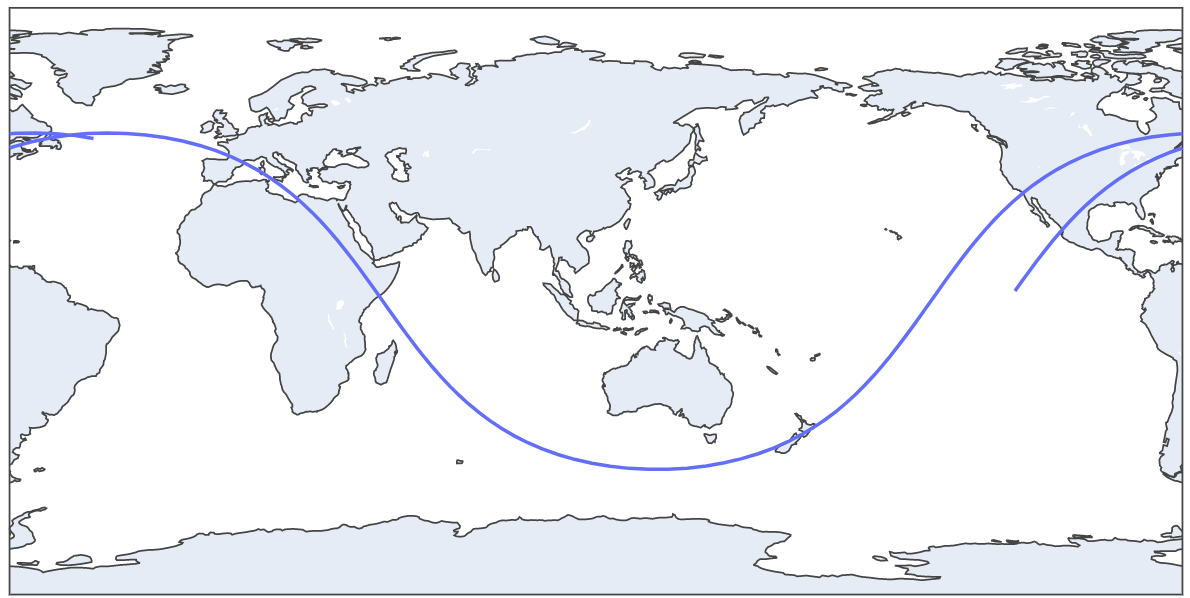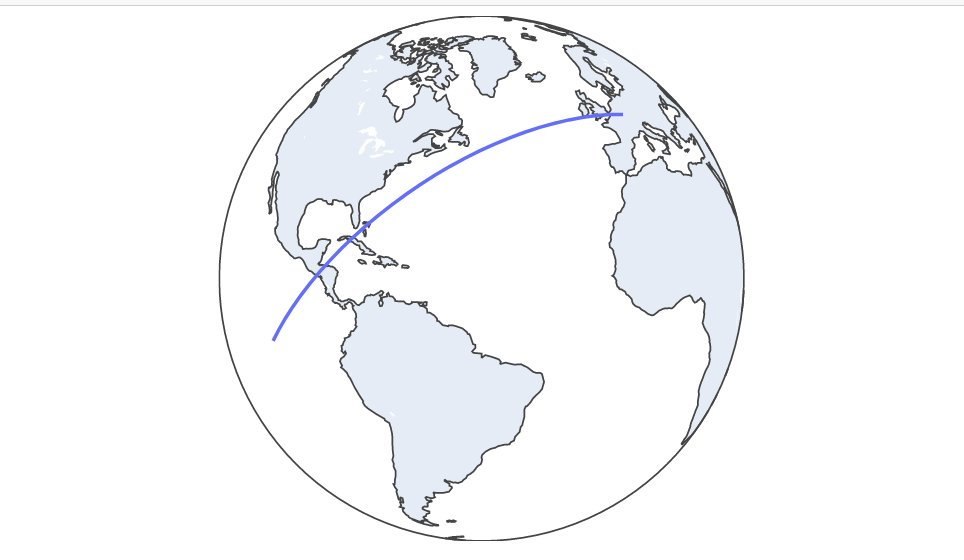# International Space Station data with Python research🌎

### Plotting trajectory:

##### We will use an API to retrieve ISS current position in latitude and longitude:

http://open-notify.org/Open-Notify-API/ISS-Location-Now/

#### First we need to import the following python modules:

###### Pandas to read json data from ISS API, plotly to make the plot of the trajectory and time to time.sleep function

```import pandas as pd
import plotly.express as px
import time```

#### Second we must initialize the list that will preserve the latitude and longitude points (every sixty seconds). You also have to initialize the N variable with time in minutes

```latitudes = []
longitudes = []
N = 60 # Sixty for one hour trajectory```

#### Then we will create the following for loop to keep recording latitude-longitude points separated by one minute

##### We use for i in range(N), which is the time that the script will keep running (in hours) because we have a time.sleep(60) at the end

```for i in range(N):
url = "http://api.open-notify.org/iss-now.json" # API URL

latitudes.append(df["iss_position"]["latitude"])  # We append latitude ISS position to latitudes list
longitudes.append(df["iss_position"]["longitude"]) # We append longitude ISS position to longitudes list

time.sleep(60) # This is used to separate de point records with one minute```

#### When the for loop finish the iterating we will have a record of N minutes ISS trajectory. Now we can plot this with Plotly (px.line_geo):

##### px.line_geo will create a plot with earth map

```fig = px.line_geo(lat=latitudes, lon=longitudes) # Passing our latitudes and longitudes list as parameter
fig.show()  ```#### We can update our plot to orthographic projection with this code:

```fig.update_geos(projection_type="orthographic")
fig.update_layout(height=300, margin={"r":0,"t":0,"l":0,"b":0})
fig.show()  ```###### 30 minutes trajectory plot### Estimating ISS velocity:

#### First import the following python modules

```import pandas as pd # Pandas to read API data
import time # Time for time.sleep
import geopy.distance # Geopy to get distance between two lat-lon points
import requests # Get another API data
import json # Read that data```
##### We need to initialize two empty list to save latitudes and longitudes

```lat = []
long = []```
##### Next we will use a for loop to get the two latitude-longitude points separated by 60 seconds (time.sleep(60))

```for i in range(2):  # for in range(2) because we want two lat-lon points

url = "http://api.open-notify.org/iss-now.json" # API url

lat.append(df["iss_position"]["latitude"]) # Append latitude to lat list
long.append(df["iss_position"]["longitude"]) # Append longitude to long list

time.sleep(60) # Wait 60 seconds to record the second lat-lon point```

#### Then we must get the distance between this points:

##### We create the two different points. The first one with lat index and long. The second one with lat and long

```coords_1 = (lat, long)
coords_2 = (lat, long)```
##### Then calculate distance with geopy library:

```distance = (
geopy.distance.distance(coords_1, coords_2).m
) # Distance between the points in meters```
##### But we must make a litle correction. Because ISS isn’t moving in earth surface. It’s orbiting aproximately 400Km above earth surface. So the radius is greater. The distance traveled is a litle bit more. To do this, we need to get ISS current altitud. Use the following code:```iss_alt_url = "https://api.wheretheiss.at/v1/satellites/25544"
r = requests.get(iss_alt_url)
r = r.text

iss_alt = int(r["altitude"]) # IN KM```
##### Now apply phisics formula to make the correction

```earth_radius = 6371 # in KM
##### Now finish the calculation with speed formula already explained:

```speed = distancia_corrected/60

print(round(speed*3.6, 3), "KM/H") # Multiplied by 3.6 to convert from m/s to km/h. Rounded by 3.```

#### Output:

``````26367.118 KM/h
``````

View Github